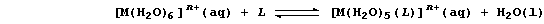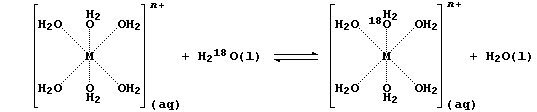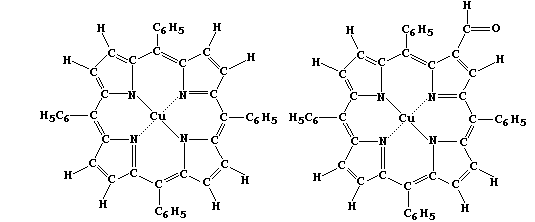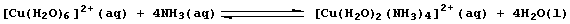```METALS: LIGAND-EXCHANGE REACTIONS
A ligand-exchange reaction is the chemical change which occurs when one
ligand is replaced by another; a ligand (L) is a molecule or an ion
(coordinatively) bonded to a central atom or ion. This type of reaction
is exemplified by reactions summarized by this general equation:``````Hydrated metal ions, formed when an ionic compound dissolves in water,
can be represented either as Mn+(aq) or as [M(H2O)6]n+(aq) - because
each metal ion is usually coordinated to six water molecules acting as
ligands. The rates of ligand-exchange between coordinated and solvent
water molecules have been investigated using labelled water; i.e.,``````No attempt is made here to summarize the extensive research directed at
measuring and explaining the rates of these particular ligand-exchange
reactions; suffice to note that, as this order illustrates, .. Pb(II) >
K(I) > Cu(II) > Na(I) > Ca(II) > Zn(II) > Fe(II) > Mg(II) > Al(III) ..,
these rates do not correspond to the metal reactivity series.
[.. K > Ca > Na > Mg > Al > Zn > Fe > Sn > Pb > (H) > Cu > Hg > Ag ..]
1.  Most molecules in living organisms are structurally very complex;
accordingly, in order to provide insight into some of their biological,
chemical, and physical properties, scientists often study simpler or
'model' compounds. Shown below are the structural formulae of two such
model compounds, which are readily prepared in two or three steps from
inexpensive reactants.``````In each compound, the four nitrogens coordinated to the Cu(II) ion form
a square plane. Suggest the geometry of the compounds formed when:
A ligand is added above the plane. ____________________________________
Ligands are added above and below the plane. __________________________

Suggest what metal ion should replace Cu(II) in the above compounds for
either to be considered as a (marginally acceptable) model for studying
ligand-exchange reactions in:
Chlorophyll-b __________ Haemoglobin __________ Vitamin-B12 ___________
```

```2.  Probably the most familiar example of ligand-exchange reactions is
that observed in the standard test for the hydrated copper(II) ion with
(excess) aqueous ammonia; formation of the characteristic 'royal-blue'
solution is usually summarized as:``````(a) Colour is an example of a qualitative variable; i.e., one where the
data are non-numerical or purely descriptive. In investigations, such a
variable can present problems in description (and, subsequently, in
interpretation), because of the inevitable element of personal opinion;
e.g., all ions corresponding to the formula [Cu(H2O)x(NH3)y]ý+(aq) are
shades of blue, and the [Cu(H2O)2(NH3)4]ý+(aq) ion has been variously
described as dark-blue, deep-blue, royal-blue, and indigo. Fortunately,
providing a suitable instrument is used, colour can be expressed as a
quantitative variable; i.e., one where the data are collected either by
counting or by measuring. Complete the Table below, by calculating the
frequency of the photon absorbed by each ion, using the formula n = fl,
where: n is the speed of light (3 æ 108 ms-1); f is the frequency; and
l is the wavelength.```
 ` Aqueous ion Colour` ``` Wavelength of photon absorbed (l) / æ 10-9 m``` ``` Frequency of photon absorbed (f) / æ 1014 Hz``` ` [Cu(H2O)6]2+ blue ` ` 820 ` ` ` ` [Cu(H2O)5(NH3)]2+ ?-blue` ` 740` ` ` ` [Cu(H2O)4(NH3)2]2+ ?-blue` ` 675` ` ` ` [Cu(H2O)3(NH3)3]2+ ?-blue` ` 630` ` ` ` [Cu(H2O)2(NH3)4]2+ ?-blue ` ` 585` ` ` ` [Cu(H2O)(NH3)5]2+ ?-blue` ` 635` ` `
```(b) The symbol equation above summarizes a series of reversible ligand-
exchange reactions that involve the successive replacement of four
molecules of water with those of ammonia. Construct the symbol equation
for one of these reversible ligand-exchange reactions. ________________
_______________________________________________________________________

For a series of reversible reactions, using Le Chatelier's Principle to
predict the overall position of equilibrium is appropriate but not
straightforward. Usually, in investigating such series, the first step
is to measure various physical quantities; e.g., the concentrations of
reactants and products. Calculate the concentration of pure water. ____
_______________________________________________________________________
_______________________________________________________________________

(c) In attempting to extend the scope of any given reaction, scientists
often consider variations (or develop hypotheses) based on the patterns
inherent in the Periodic Table; e.g., variants of Cu(II), H2O, and NH3
might reasonably include Ag(II), H2S, and PH3, respectively. An equally
rich source of variation lies in the patterns of organic chemistry ...
Each homologous series can be represented by a general formula; e.g.,
CnH2n+2 for chain alkanes. The so-called 'trivial' member of a series
is the compound whose formula corresponds to n = 0; this is dihydrogen
for the alkanes, whose first true member is methane. Several members of
an homologous series, which contains ammonia as the trivial member, act
as ligands in similar exchange reactions. Determine the general formula
of this series, and draw the structural formulae of its first two true
members. ______________________________________________________________
_______________________________________________________________________
_______________________________________________________________________
_______________________________________________________________________
_______________________________________________________________________
_______________________________________________________________________

Dr. R. Peters                   Next                     Contents' List```